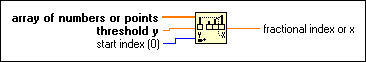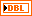# Threshold 1D Array Function

## LabVIEW 2018 Help

Edition Date: March 2018
Part Number: 371361R-01
View Product InfoLabVIEW 2016 HelpLabVIEW 2017 HelpLabVIEW 2018 HelpLabVIEW 2019 HelpLabVIEW 2020 Help

Owning Palette: Array Functions

Requires: Base Development System

Interpolates points in a 1D array that represents a 2D non-descending graph. This function compares threshold y to the values in array of numbers or points starting at start index until it finds a pair of consecutive elements such that threshold y is greater than or equal to the value of the first element and less than or equal to the value of the second element.

The connector pane displays the default data types for this polymorphic function.array of numbers or points can be an array of numbers or an array of points where each point is a cluster of x- and y-coordinates. If this input is an array of points, this function uses the second elements in the clusters, or the y-coordinates, to obtain a fractional index that it then uses to interpolate the corresponding x value.threshold y is the threshold value for the function. If threshold y is less than or equal to the array value at start index, the function returns start index for fractional index or x. If threshold y is greater than every value in the array, the function returns the index of the last value. If the array is empty, the function returns NaN.start index must be a number. The default is 0, which means the function returns the result calculated from the entire array, rather than a specified section of the array.fractional index or x is the interpolated result LabVIEW calculates for the array of numbers or points 1D input array. For example, suppose array of numbers or points is an array of four numbers [4, 5, 5, 6], start index is 0, and threshold y is 5. The fractional index or x is 1, corresponding to the index of the first value of 5 the function finds. Suppose the array elements are 2.3, 5.2, 7.8, 7.9, 10.0, the start index is 0, and the threshold y is 6.5. The output is 1.5 because 6.5 is halfway between 5.2 (index 1) and 7.8 (index 2). If threshold y is 7 for the same set of numbers, the output is 1.69. If threshold y is 14.2, start index is 5, and the values in the array starting at index 5 are 9.1, 10.3, 12.9, and 15.5, threshold y falls between elements 7 and 8 because 14.2 is midway between 12.9 and 15.5. The value for fractional index or x is 7.5, that is, halfway between 7 and 8. If the array input consists of an array of points where each point is a cluster of x- and y-coordinates, the output is the interpolated x value corresponding to the interpolated position of threshold y among the y-coordinates, rather than the fractional index of the array. If the interpolated position of threshold y is midway between the y values at indexes 4 and 5 of the array with x values of –2.5 and 0 respectively, the output is not an index value of 4.5 as it would be for a numeric array, but rather an x value of –1.25. In other words, this function returns the interpolated x value associated with the given y value if you graphed the points. This function works the same for arrays of numbers as it does for arrays of points. If you have an array of numbers, this function assumes the x-coordinates are the same as the indexes of the array itself. In other words, this function assumes the points are uniformly spaced.

## Threshold 1D Array Details

This function calculates the fractional distance between the first value and threshold y and returns the fractional index at which threshold y would be placed within array of numbers or points using linear interpolation.Note  Use this function only with arrays sorted in non-descending order.

This function does not recognize the index of a negative slope crossing, and it might return incorrect data if threshold y is less than the value at start index. Use the Threshold Detector VI for more advanced analysis of arrays.